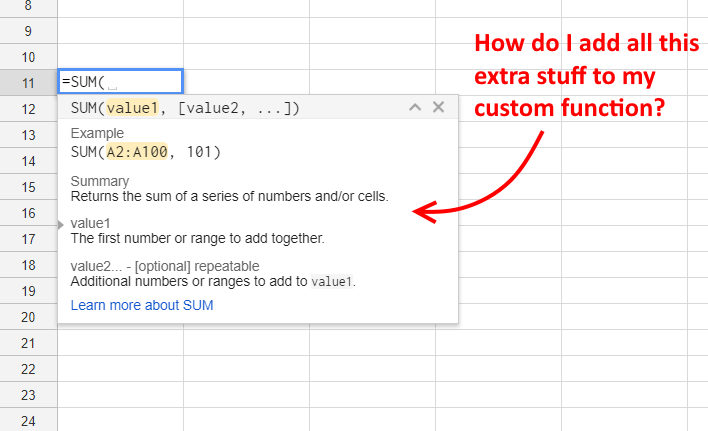## Google Sheets – How to Separate the First Name from a Full Name Cell

left, right, find, length

Every academic quarter I receive a list of students by their full name in one cell that I need to split into a cell for the first name and then a cell for the middle and last names combined.

This fairly simple process can be achieved with the Google Sheets formulas left, right and find.

If you are in a hurry, here are the formulas below:

## First Names

To get the first name we do the following:

=LEFT(A2,FIND(” “,A2))

Where ‘A2’ is the cell that we have our full name in – in our case Vasco Nunez de Balboa.

When you have multiple formulas in a cell it’s often best to go from the inside out. So let’s first look at what FIND does.

FIND looks inside the cell for the first value that we want to search for. For us, it’s an empty space, ” “. Find then returns the numerical position of that found item.  To do this FIND takes two arguments:

=FIND(the item we are searching for, the cell or string the item is in)

For example, if we are searching for the location of the space in
Vasco Nunez de Balboa  which is in cell A2,  we would do the following:

=FIND(” “,A2)

Which would give the result: 6

There are five letters in the first name Vasco. The space would be in position 6.

Now that we have the position of the space, lest just grab everything in the cell to the left of that space. We do this with LEFT

LEFT also take two values. The first is cell location and the second is the number of characters we want to take from the left-hand side.

LEFT(cell location, number of characters from the left)

Now that we know the first space is character 6, the formula would look like this:

LEFT(A2,6)

We then replace the 6 with our FIND formula and we are good to go.

## Google Apps Script – How to Get the Sheet Name and Spreadsheet Name and add to a Cell on Google Sheets with a Custom Function

Boy, are these titles getting longer.

But that’s pretty much the gist of it. In this post we will look at creating a Google Apps Script Custom Function that allows you to do Three things in Google Sheets:

1. Get the current sheet name.  That’s the same sheet name as the cell you are working in.
2. Get all the sheet names. A full list of all the sheet names.
3. Get the name of the Spreadsheet file.

The above picture is pretty self-explanatory. If you type in:

=SHEETNAME(#)

Where “#”  is a number 0, 1 or 2 you will get the results displayed in the picture. Any other number will display an error.

## Google Sheets – Remove The Lowest Grade for Each Student on a Course

Google Sheets – MIN, FILTER, INDEX, MATCH, SUM, COUNTIF

In the region of the world that I work in, it is a pretty common occurrence for university courses to run weekly assessment. At the end of the course, all the weekly assessment is then added together minus the lowest piece of assessment.

For lecturers with small course sizes, this is a pretty simple task that you could simply eyeball if you have a small enough group, but what if your course runs into the thousands with half a dozen tests to choose from. Eyeballing is just not going to do it.

Recently I was asked to do the same thing for the program that I manage. Over an 8 week term, we run 7 assessment at the end of each week for our students.  My job was to find the lowest grade out of the 7 assessment and drop it, taking note of the assessment unit that I dropped for each student.

I use Google Sheets for this purpose for its ease of use and sharability.

This is an example dataset of the 7 assessment (in this case, weekly tests) in Google Sheets. We need to remove the lowest grade from each student. As you can see not all students have their lowest grade in the same Unit test.

## Google Sheets – Get the Top 2 Grades for Each Group in a Spreadsheet using RANK and FILTER

This week I was asked to provide a list of the top two student grades in each of the 100 classes in our university program.

Normally, this would be a pretty easy task if there were only a half dozen or so classes. Simply sort by group (class) and then Grade. Finally, copy and paste the top 2 ranked students of each group in a new sheet – print and hand to the boss.

However, with 100 classes this was going to turn into a time-consuming chore and one that could be prone to errors.

To solve this problem, I took advantage of Google Sheets RANK.EQ and FILTER formulas. Let’s take a peek at the formula now and go through the steps in detail later.

Let’s move on to an example.

## Google Apps Script – How to make a Custom Function to Use in Google Sheets